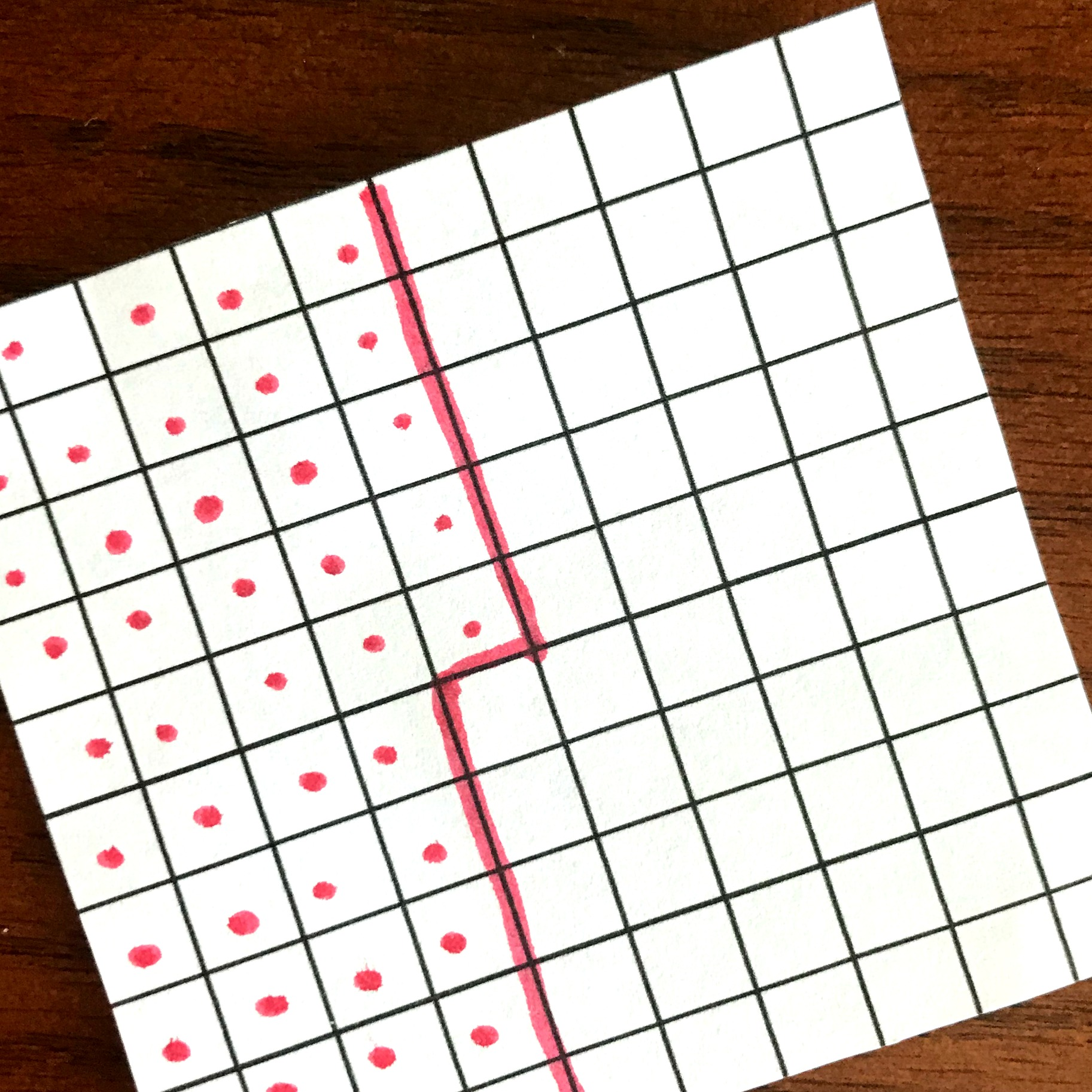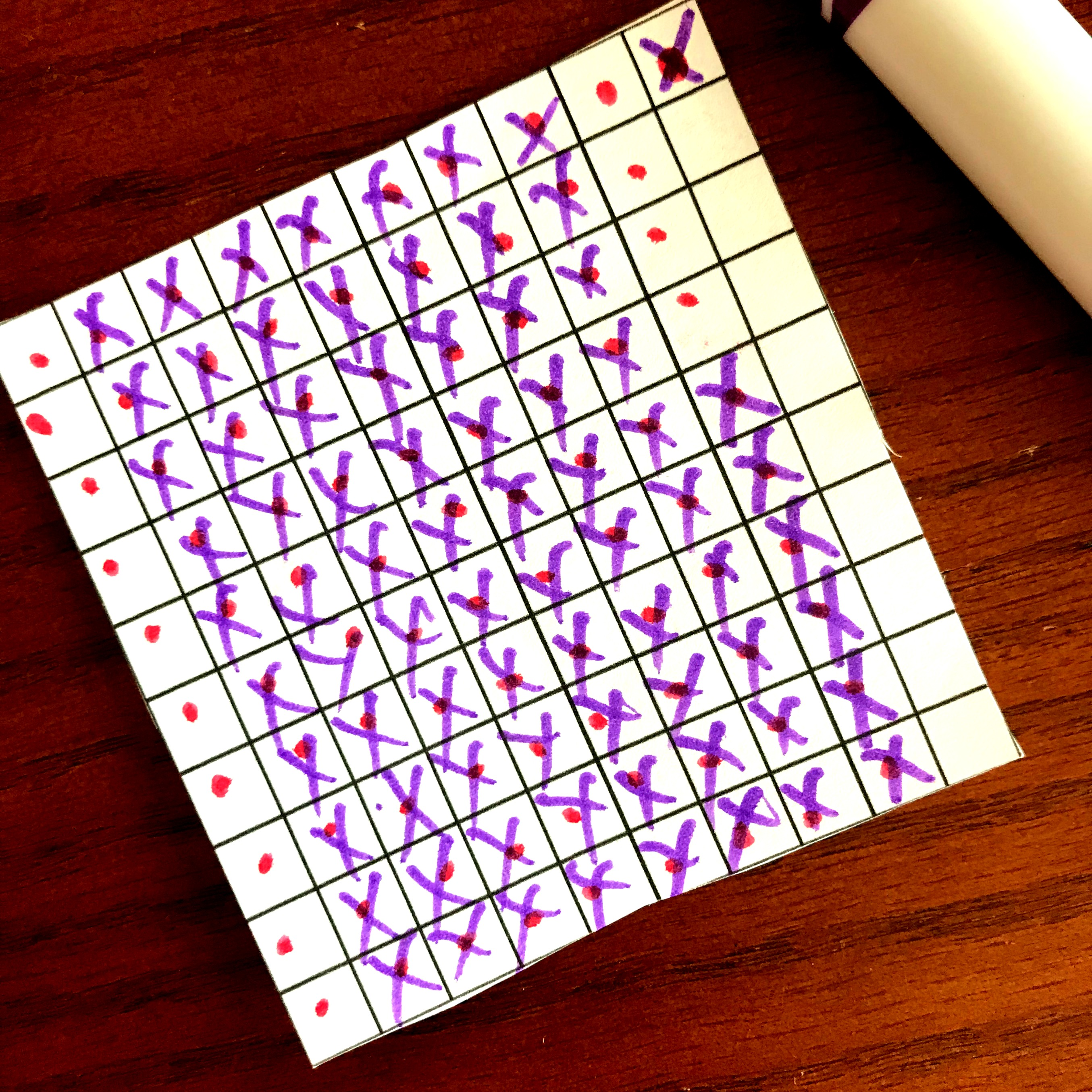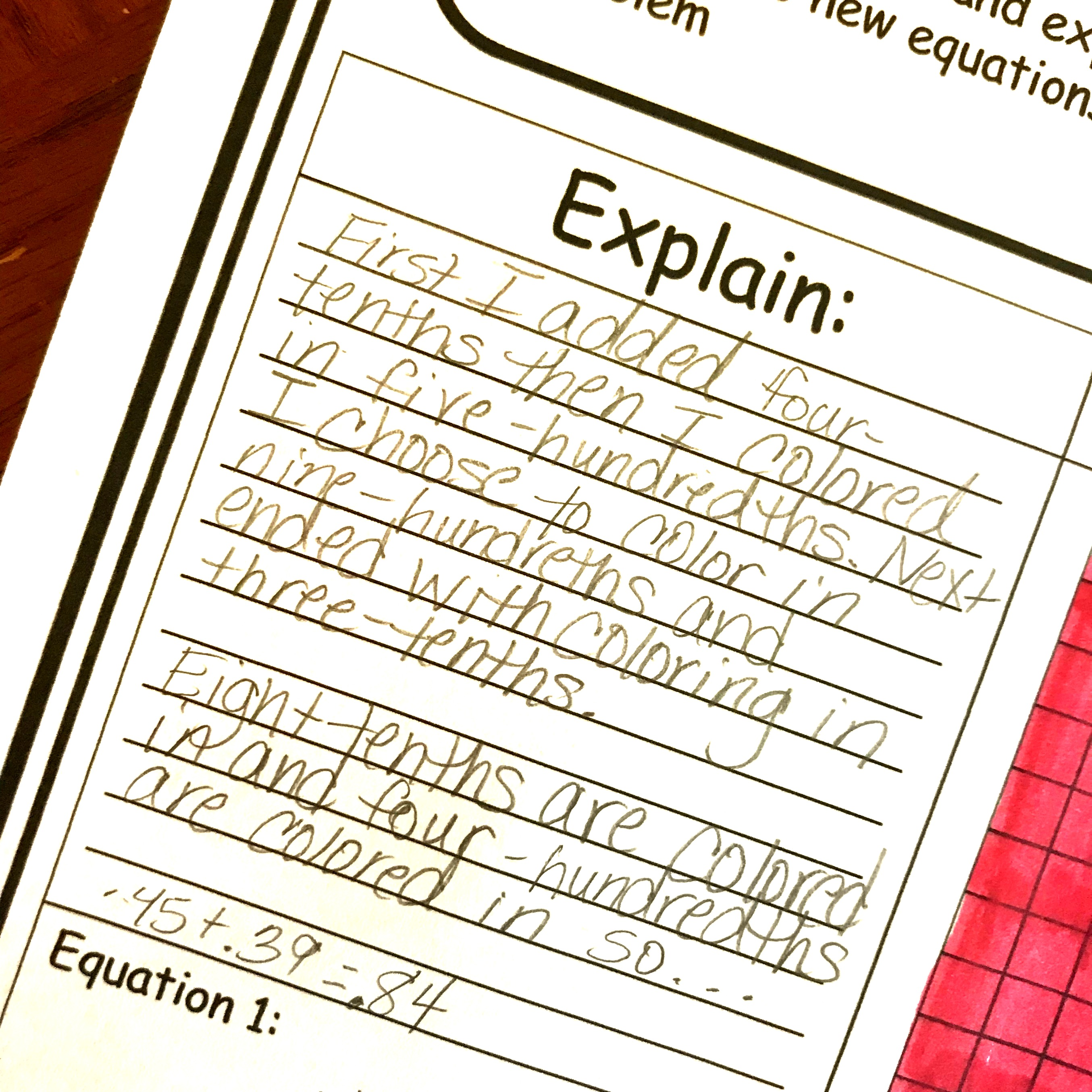Home » Math » FREE Decimal Addition and Subtraction Worksheets Using Decimal Grids

FREE Decimal Addition and Subtraction Worksheets Using Decimal Grids

These decimal addition and subtraction worksheets get children modeling and explaining how they solved a problem, and creating new equations.You can get this FREE download by clicking on the blue button at the VERY end of the post.

This month, Bethany from Math Geek Mama and I have been exploring different ways to add and subtract decimals. ANd this is what we have for you:

First, there is adding and Subtracting with Base Ten Blocks File Folder Activity

And we move into Adding and Subtracting WIth Base Ten Blocks Game

Next, there is Adding and Subtracting with Number Lines

And today, our post is how to adding and subtracting with decimal grids.

The final post is practice for Adding and Subtracting With Number Lines and Decimal Grids

So here we go…..how do you add and subtract decimals on a decimal grid???

Let’s say we have the problem, .45 + .32. This is simple to solve on a decimal grid. First, we have to color in .45. If your children are familiar with decimals they know that the four represents 4 tenths. We can color in a decimal grid (which is 100 squares) to represent 4 tenths, by coloring in four out of the ten longs.

Now we move unto the five hundredths Once again this is easy to color in. Now we color in five of the little squares because five-hundredths is five out of the hundred squares on the grid.To finish off the problem, we can have to add 3 tenths and 2 hundredths.Finally, we add up all that we have colored in. This is Seven tents and seven hundredths so my answer is .77.

(If it makes it easier on your child, you could add all the tenths first, and then add all hundredths. This way the tenths will be together and the hundredths together. It may make it easier for them to add up.

Subtracting:

Subtracting, even when regrouping is required, is incredibly simple on a decimal grid.

Simply begin by coloring in the first number of the subtraction problem. In this example, .91 – .57, we will color in nine-tenths and 1 hundredths.Next, we get to do some crossing off. We cross off seven hundredths, and then five tenths.What we have left is .14!!!!

Decomposing:

Decomposing numbers is a wonderful way to build number sense. And doing this with decimal grids is easy because it is very visual to see.

Let’s look back at our addition problem. How else could we write this?

Well, I see that I have four-tenths colored in and the three-tenths colored in. Then there are two hundredths and five hundredths. So I could write out my problem as .4 + .3 + .05 + .02 = .77

It is actually easier to add that way, and all the while you are building place value sense which goes a long way in helping children be successful in math.

Prep-Work:

Once children have had a chance to play with adding and subtracting on decimal grids under your direct instruction, now it is time to move on to the worksheets. And there is no prep.

1. First, print off the worksheets.
2. Then gather up colored pencils and pencils and you are done.

decimal addition and subtraction worksheets

Decimal Addition and Subtraction Worksheets

Now it is totally up to your kiddos to take what you have shown them and prove how well they can add and subtract decimals.

On each sheet, they are given one problem to solve. Their first step should be to solve using the decimal girds.Next, comes the fun part. Writing it out. It is important that children write in math, and this gives them that chance. And all they need to do is tell what they did to solve the problem.Finally, they are to create two equations using the grids!And that is it. Your children are exploring decimals, decomposing them, adding and subtracting, modeling, and explaining their thinking using math vocabulary…..all in one simple activity.

You’ve Got This,

Rachel

You May Also Like:

Our complete adding and subtracting bundle using base ten blocks.